# How Infinite Series Convergence Explains Swinging Motion Of A Pendulum?

###### ByRamraj Saini###### Synopsis

You know those things that swing like the pendulum in old clocks? Do you know maths can help us understand their motion? This article talks about pendulum movement, using simple maths like small-angle tricks, infinite series, and the Binomial Theorem, and why it's important for learning. Read on to learn more.###### Synopsis

You know those things that swing like the pendulum in old clocks? Do you know maths can help us understand their motion? This article talks about pendulum movement, using simple maths like small-angle tricks, infinite series, and the Binomial Theorem, and why it's important for learning. Read on to learn more.

Pendulums, those things that swing back and forth like the ones on old clocks, have always fascinated people. They can be found in many places, from Grandpa's clock to machines that detect earthshakes. But have you ever wondered how we use maths to understand and describe their swinging? Believe it or not, maths comes to the rescue in the form of something called infinite series, sequences, and the Binomial Theorem. In this simple article, we'll explore these maths ideas and see how they help us understand the way a pendulum moves.

## What Is The Pendulum?

Before we dive into the maths stuff, let's talk about what makes pendulums so cool. A pendulum is just a weight (imagine a small ball) hanging on a string or rod, and it swings back and forth.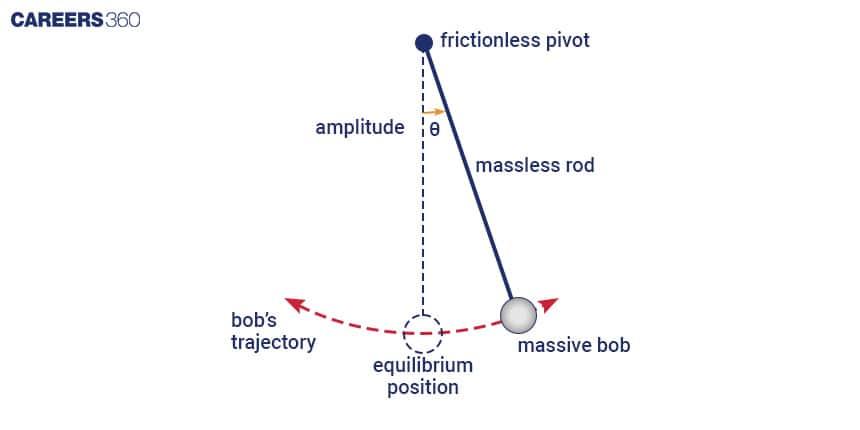This swinging happens because of two important forces:

>> Gravity: It's what pulls the weight down when it's away from the middle.

>> Tension: The string or rod tries to pull the weight back to the middle.

Together, gravity and tension create the back-and-forth swing we love to watch. Now, let's see how maths helps us understand this.

## The Simple Pendulum Equation

We want to use maths to describe how a pendulum swings. The way we do it is by using an equation. The equation looks like this:

d2θ/dt2 + (g/L)sin(θ) = 0

The letters might look intimidating, but they stand for simple things:

• θ is the angle of the pendulum from straight up.

• t is time.

• g is how fast things fall because of gravity.

• L is how long the pendulum is.

This maths tells us how the pendulum moves. It's a bit like a recipe for making a cake. Now, let's talk about sequences and how they make things simpler.

## Sequences And Easy Angles

Sequences are like lists of numbers. They help us understand how the angle of the pendulum (θ) changes as it swings. To make things simpler, we use something called the "small-angle approximation." It's like saying the angle is not too big. When the angle is small (less than 20 degrees), we can make our equation much simpler:

sin(θ) ≈ θ

Now our equation becomes easier:

d2θ/dt2 + (g/L)(θ) = 0

This simpler equation helps us understand how the pendulum swings when the angle is not too big. But how do we get this "sin" and "θ" thing? This is where infinite series come into play.

## Understanding Convergence

In maths, we often use something called infinite series. These are like never-ending lists of numbers. Convergence is when the numbers in the list get closer and closer to a specific value.

The cool thing is that the "sin" function can also be written as an infinite series. When we use this series, we can get a good idea of how the pendulum moves. But here's the catch: the series works well for small angles. As the angle (θ) gets bigger, the higher-number terms in the series become important, and the list doesn't get closer to a single value as quickly. This is where the idea of a "convergence radius" comes in. It's like a limit for how big the angle can be.

For the "sin" function, this radius is really big, meaning the series works well for most angles. But for really big angles, we need to use other maths tricks.

## Binomial Theorem: Another Helpful Trick

The Binomial Theorem is like a handy tool in our maths toolbox. It helps us simplify things when the value is small. It works like this:

(1 + x)n = 1 + nx + [n(n-1)/2!](x2) + [n(n-1)(n-2)/3!](x3) + ...

For small values of x, higher-order terms of x can be neglected, and we can now express this as:

(1 + x)n ≈ 1 + nx

For small values, we use this trick to simplify the "sin" function:

cos x = 1 - x2/2! + x4/4! - x6/6!+...

sin x = x - x3/3! + x5/5! - x7/7!+...

sin(x) ≈ x

Now our maths is easy to work with when the value is not too big.

## Time For the Pendulum's Beat

One of the most important things about a pendulum is how fast it swings back and forth, called its "time period." let's derive it with maths.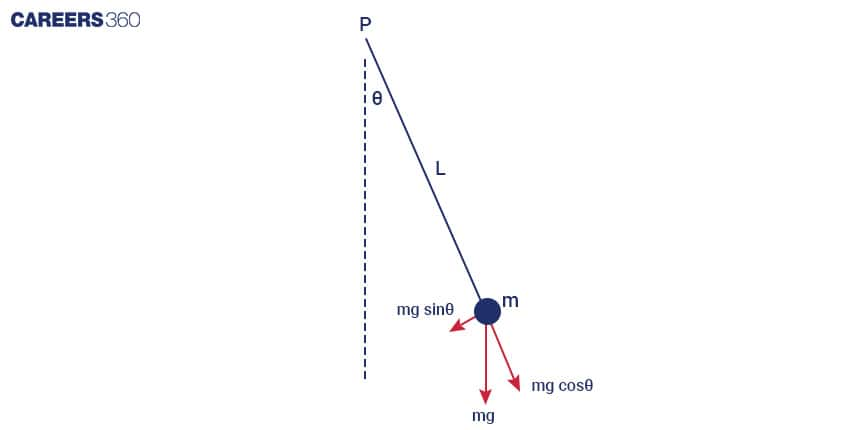A basic pendulum involves a point-mass ball (m) suspended from a weightless string of length (L) and anchored at a pivot point (P). When it's moved to an initial angle and then let go, the pendulum swings back and forth in a repeating pattern. Using Newton's second law for rotational systems, we can derive the equation that describes how the pendulum moves

τ = Iα

-mgsin θ L = mL2d2θ/dt2

For small angle sin(x) ≈ x

mL2(d2θ/dt2) + mg θL = 0

d2θ/dt2 + (g/L) θ= 0

The simple harmonic solution for this equations is

t) = θ0cos(ωt)

ω = √(g/L)

T = 2π/ω = 2π √(L/g)

The period (T) for a simple pendulum is given by this simple formula:

T ≈ 2π √(L/g)

What's cool is that this formula doesn't matter how big the angle is; it only depends on the length of the pendulum (L) and the strength of gravity (g).

## When Accuracy Matters

While our simple maths works well in most cases, sometimes we need to be more precise. For important things like keeping time or measuring earthquakes, we can't always rely on the small-angle approximation. In those cases, we use more advanced maths, computers, and special techniques to describe how the pendulum really moves, no matter how big the angle is.

## Value For Students

Now, you might be wondering, Why is all of this important? Well, for students, learning about how maths is used to understand the motion of pendulums isn't just about the swinging metal ball.

>> Problem-Solving Skills: It helps develop your problem-solving skills. You learn how to take a complex situation and break it down into manageable parts.

>> Real-World Applications: Understanding maths in real-life situations is valuable. You see how maths isn't just numbers on paper; it's a tool to understand the world around us.

>> Applying Theory: You get to apply mathematical theory to practical situations. This is where maths truly comes to life.

>> Preparation for Advanced Study: If you ever decide to pursue a career in physics, engineering, or any science-related field, this foundation is crucial.

And for those students who are wondering where these concepts are discussed in their NCERT textbooks, you'll find them in your physics and mathematics books. The concepts of sequences, series, and trigonometry, which are fundamental for understanding pendulum motion, are often introduced in class 11 and 12 textbooks.

So, next time you see a pendulum swinging or you're working through maths problems in class, remember that maths isn't just about numbers; it's about understanding the world and preparing you for the future. It's all about learning how things work, and pendulums are just one example of how maths adds value to your education.

## Careers360 helping shape your Career for a better tomorrow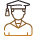#### 250M+

Students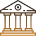#### 30,000+

Colleges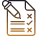#### 500+

Exams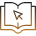E-Books